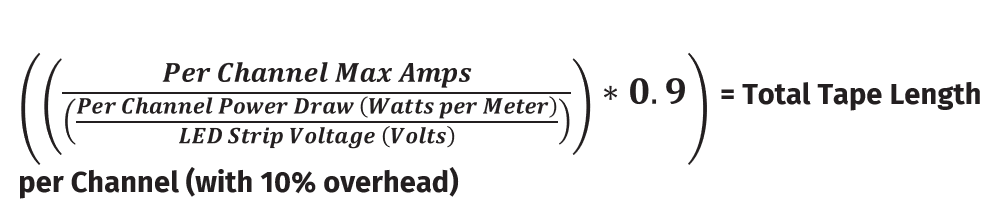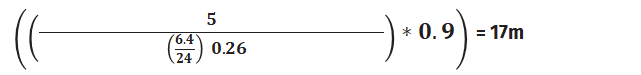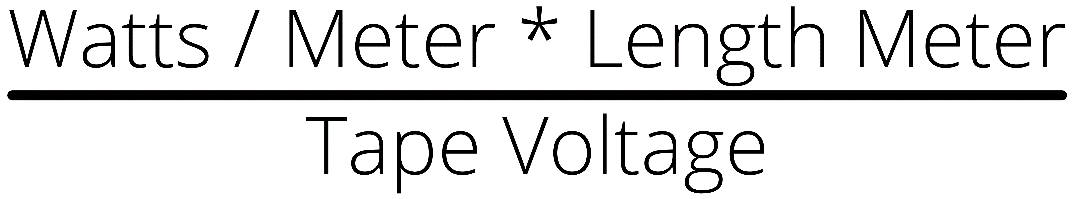The CVC4 LED Dimmer can dim a total capacity of 20A per unit between 12-24 Volts DC, with each channel rated up to 5A.

Always follow your LED strip manufacturer's recommendations for maximum single run length.

### To calculate the maximum length of tape a CVC4 can control:

We use the following calculation:1. Identify the channel with the highest power consumption (Watts) per Meter. By using the highest value, we ensure that no channel goes above 5 Amps and the total does not exceed 20 Amps.
2. Divide this channel's Watts per Meter value with the LED Strip Voltage. This will give you the Amps per Meter.
3. Divide the per channel max Amps (5) by this.
4. Multiply this by 0.9 to protect your installation by allowing a 10% overhead to factor in inrush current.

Example:

 24V RGBW TAPE R G B W Watts / Meter 4.45 3.31 3.12 6.41. The White (W) channel has the highest Watts per Meter
2. Divide this channels Watts per Meter (6.4) with the LED Strips Voltage (24) = 0.26
3. Divide the per channel max Amps (5) by 0.26 = 19.23
4. Multiply 19.23 by 0.9 to protect your installation by allowing a 10% overhead = 17.30

### ENTTEC Tape:

 9VLW-10 R G B W Watts / Meter 4.45 3.31 3.12 6.49VL-10 R G B Watts / Meter 4.45 3.31 3.12### To calculate the current draw of your tape:1. Multiply watts per meter by the length in meters. (follow manufacturer recommendation's for maximum single run length)
2. Divide total wattage by the voltage of the tape. This will give you the Amps.

Current (Amps) must be calculated for each channel on the tape. For example, R, G, B, W. The channel with the highest current will determine the maximum length of the tape.

In the example below, all 4 channels are under 5 Amps, and the total does not exceed 20.

 24V RGBW TAPE Watts / Meter Length (M) Total Watts Amps / Channel R 4.45 10 44.5 1.85 G 3.31 10 33.1 1.38 B 3.12 10 31.2 1.3 W 6.4 10 64.0 2.66

In the example below, channel 4 is 5.3 Amps, which exceeds the 5 amp maximum per channel. The total length of the tape would need to be reduced so that channel 4 is 5 Amps or less.

 24V RGBW TAPE Watts / Meter Length (M) Total Watts Amps / Channel R 4.45 20 89 3.7 G 3.31 20 66.2 2.76 B 3.12 20 62.4 2.6 W 6.4 20 128 5.3